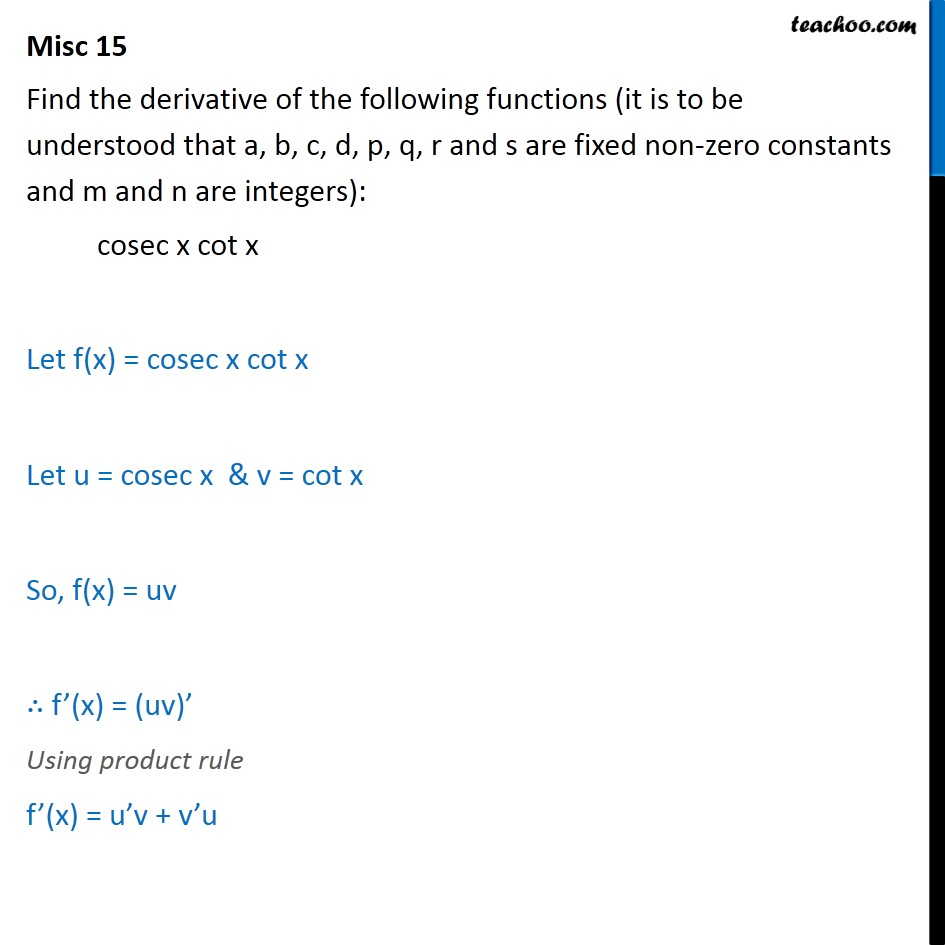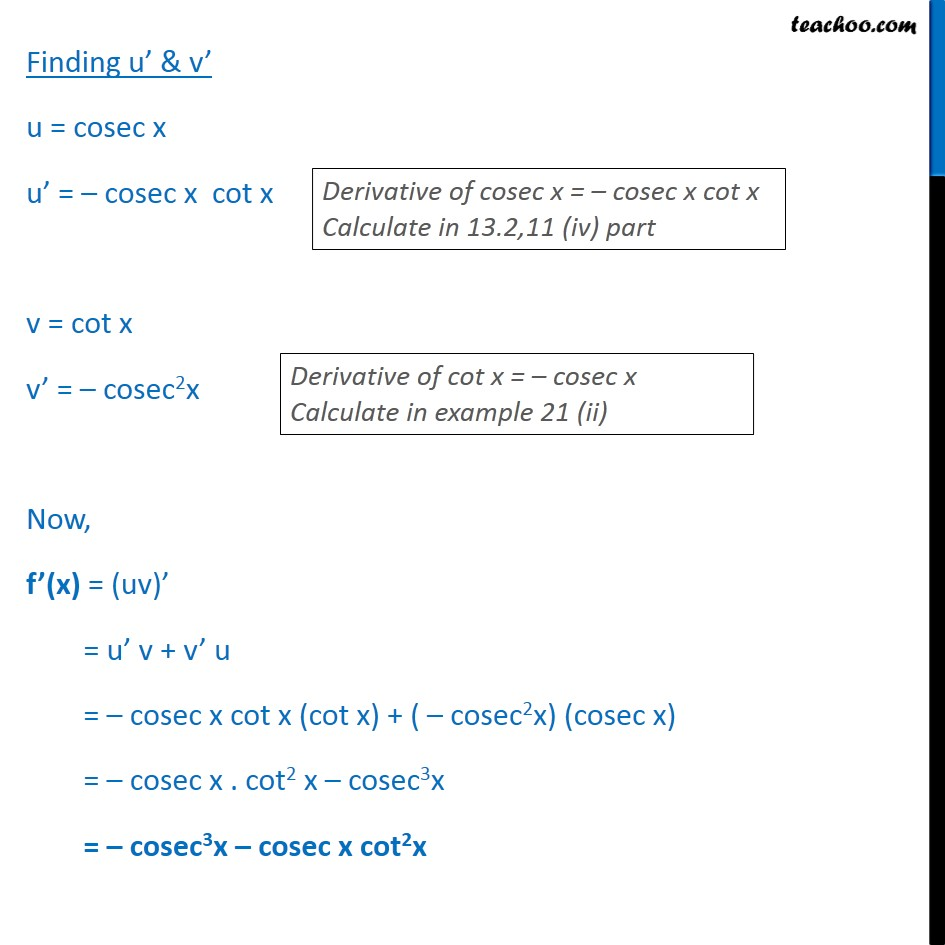1. Chapter 13 Class 11 Limits and Derivatives (Term 1 and Term 2)
2. Serial order wise
3. Miscellaneous (Term 1 and Term 2)

Transcript

Misc 15 Find the derivative of the following functions (it is to be understood that a, b, c, d, p, q, r and s are fixed non-zero constants and m and n are integers): cosec x cot x Let f(x) = cosec x cot x Let u = cosec x & v = cot x So, f(x) = uv f (x) = (uv) Using product rule f (x) = u v + v u Finding u & v u = cosec x u = cosec x cot x v = cot x v = cosec2x Now, f (x) = (uv) = u v + v u = cosec x cot x (cot x) + ( cosec2x) (cosec x) = cosec x . cot2 x cosec3x = cosec3x cosec x cot2x

Miscellaneous (Term 1 and Term 2)